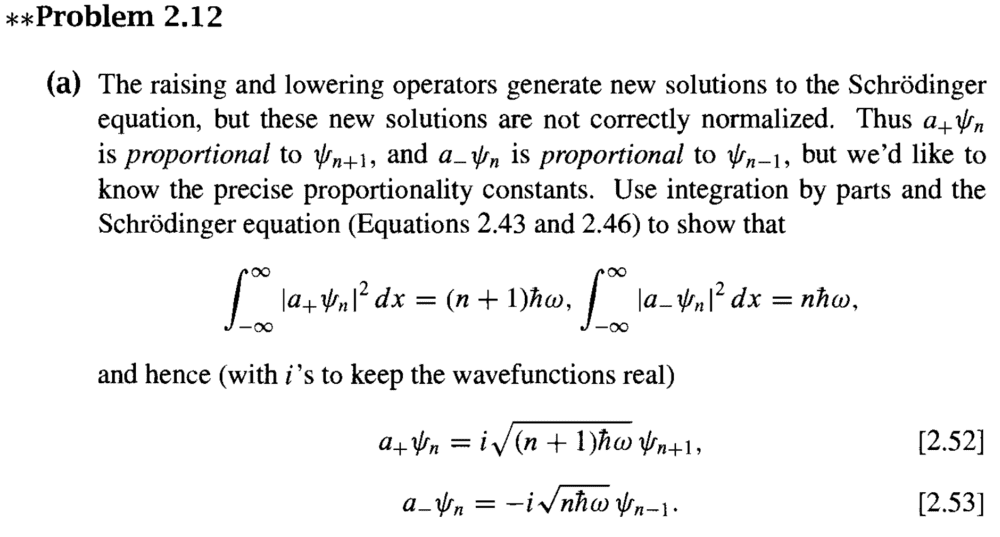# Normalisation constants with ladder operators

etotheipi
Homework Statement:
The wave function of a particle in a harmonic potential is an expansion of terms of the form$$\psi_n(x) = A_n (a_+)^n e^{-\frac{m\omega}{2\hbar }x^2}$$Determine the coefficients ##A_n##

Answer: $$A_n = \left (\frac{m\omega}{\pi \hbar} \right)^{\frac{1}{4}} \frac{(-i)^n}{\sqrt{n!(\hbar \omega)^n}}$$
Relevant Equations:
N/A
The previous part was to show that ##a_+ \psi_n = i\sqrt{(n+1)\hbar \omega} \psi_{n+1}##, which I just did by looking at$$\int |a_+ \psi_n|^2 dx = \int \psi_n^* (a_{-} a_+ \psi_n) dx = E+\frac{1}{2}\hbar \omega = \hbar \omega(n+1)$$so the constant of proportionality between ##a_+ \psi_n## and ##\psi_{n+1}## will have modulus ##\sqrt{\hbar \omega(n+1)}##. Then it asks to determine the normalisation constant ##A_n##, so I looked at the lowest energy state first$$\psi_0 = A_0 e^{-\frac{m\omega}{2\hbar}x^2}$$ $$\int |\psi_0|^2 dx = |A_0|^2 \int e^{-\frac{m\omega}{\hbar}x^2} dx = |A_0|^2 \sqrt{\frac{\pi \hbar}{m\omega}} = 1 \implies |A_0| = \left (\frac{m\omega}{\pi \hbar} \right)^{\frac{1}{4}}$$where I used the result for the Gaussian integral without proof. Then I just assumed that since ##\psi## can always be taken to be real, we can just take ##A_0 = \left (\frac{m\omega}{\pi \hbar} \right)^{\frac{1}{4}}##. Then using the previous result, we would have$$\psi_n = \left( \frac{a_+}{i\sqrt{\hbar \omega(n+1)}} \right)^n \left (\frac{m\omega}{\pi \hbar} \right)^{\frac{1}{4}} e^{-\frac{m\omega}{2\hbar}x^2}$$Edit: On second thoughts, this equation is obviously incorrect, because I don't account for the fact that ##n## is different (not constant) each time we apply the operator

Last edited by a moderator:

haruspex
Homework Helper
Gold Member
2020 Award
Not an area I know anything about, but I see you need a factorial in the answer, and you have some relationship between ##a_+ψ_n## and ##ψ_{n+1}## that involves a factor n+1. This suggests finding the relationship between ##(a_+)^{n+1}ψ_0## and ##ψ_{n+1}##.

•etotheipi
etotheipi
@haruspex you are absolutely right, I realise my mistake... it should be$$\psi_n = A_n (a_+)^n e^{-\frac{m\omega}{2\hbar }x^2} = \frac{A_n}{A_0} (a_+)^n \psi_0$$and then we expand it up to ##\psi_n##, using the fact that ##a_+ \psi_0 = i\sqrt{\hbar \omega} \psi_1##, ##a_+ \psi_1 = i\sqrt{2\hbar \omega} \psi_2##, etc. so that$$\psi_n = \frac{A_n}{A_0} (i\sqrt{\hbar \omega})(i\sqrt{2\hbar \omega})\dots(i\sqrt{n\hbar \omega}) = \frac{A_n}{A_0} i^n \sqrt{n! (\hbar \omega)^2}$$

Last edited by a moderator:
PeroK
Homework Helper
Gold Member
2020 Award
The previous part was to show that ##a_+ \psi_n = i\sqrt{(n+1)\hbar \omega} \psi_{n+1}##, which I just did by looking at$$\int |a_+ \psi_n|^2 dx = \int \psi_n^* (a_{-} a_+ \psi_n) dx = E+\frac{1}{2}\hbar \omega = \hbar \omega(n+1)$$so the constant of proportionality between ##a_+ \psi_n## and ##\psi_{n+1}## will have modulus ##\sqrt{\hbar \omega(n+1)}##.

•etotheipi
etotheipi

I was... but not so much any more! The schroedinger equation in ladder operator form looks like$$(a_+ a_- - \frac{1}{2}\hbar \omega) \psi_n = E_n \psi_n$$So I said that$$\int (a_+ \psi_n)^* (a_+ \psi_n) dx = \int \psi_n^* (a_+ a_- \psi_n) dx = (E_n + \frac{1}{2}\hbar \omega) \int \psi_n^* \psi_n dx = (E_n + \frac{1}{2}\hbar \omega) = \hbar \omega (n+1)$$We are to expect that ##a_+ \psi_n = c\psi_{n+1}##, so $$\int |c|^2 |\psi_{n}|^2 dx = |c|^2$$and the modulus of the proportionality constant would be$$|c| = \sqrt{\hbar \omega(n+1)}$$

Last edited by a moderator:
PeroK
Homework Helper
Gold Member
2020 Award
I was... but not so much any more! The schroedinger equation in ladder operator form looks like$$(a_+ a_- + \frac{1}{2}\hbar \omega) \psi_n = E_n \psi_n$$So I said that$$\int (a_+ \psi_n)^* (a_+ \psi_n) dx = \int \psi_n^* (a_+ a_- \psi_n) dx = (E_n + \frac{1}{2}\hbar \omega) \int \psi_n^* \psi_n dx = (E_n + \frac{1}{2}\hbar \omega) = \hbar \omega (n+1)$$We are to expect that ##a_+ \psi_n = c\psi_{n+1}##, so $$\int |c|^2 |\psi_{n}|^2 dx = |c|^2$$and the modulus of the proportionality constant would be$$|c| = \sqrt{\hbar \omega(n+1)}$$
Usually we have:
$$a_+ \psi_n = \sqrt{n+1} \psi_{n+1}$$
You must be using something non-standard somewhere.

PS It must be the definition of ##a_+##.

•etotheipi
PeroK
Homework Helper
Gold Member
2020 Award
The previous part was to show that ##a_+ \psi_n = i\sqrt{(n+1)\hbar \omega} \psi_{n+1}##,

I haven't seen this before. Where is this from out of interest?

•etotheipi
etotheipi
I haven't seen this before. Where is this from out of interest?

Here is the original problem statement for the first part:PS It must be the definition of ##a_+##.

For trouble-shooting purposes, Griffiths has defined ##a_+## to be the operator$$a_+ = \frac{1}{\sqrt{2m}} \left( \frac{\hbar}{i} \frac{d}{dx} + im \omega x\right)$$

PeroK
Homework Helper
Gold Member
2020 Award
Here is the original problem statement for the first part:

View attachment 267474

For trouble-shooting purposes, Griffiths has defined ##a_+## to be the operator$$a_+ = \frac{1}{\sqrt{2m}} \left( \frac{\hbar}{i} \frac{d}{dx} + im \omega x\right)$$
Is that an old edition?

•etotheipi
etotheipi
Yeah, it's older than me. I wonder what the more modern definition of the ladder operators are?

PeroK
•